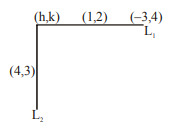Deepak Scored 45->99%ile with Bounce Back Crack Course. You can do it too!

# Suppose that the points (h,k), (1,2) and (–3,4) lie on the line

Question:

Suppose that the points $(\mathrm{h}, \mathrm{k}),(1,2)$ and $(-3,4)$ lie on the line $\mathrm{L}_{1}$. If a line $\mathrm{L}_{2}$ passing through the points $(\mathrm{h}, \mathrm{k})$ and $(4,3)$ is perpendicular to $\mathrm{L}_{1}$,'

then $\frac{\mathrm{k}}{\mathrm{h}}$ equals :

1. 3

2. $-\frac{1}{7}$

3. $\frac{1}{3}$

4. 0

Correct Option: , 3

Solution:equation of $\mathrm{L}_{1}$ is\

$y=-\frac{1}{2} x+\frac{5}{2}$     ...........(1)

equation of $\mathrm{L}_{2}$ is

$y=2 x-5$            .......(2)

by (1) and (2)

x = 3

$y=1 \Rightarrow h=3, k=1$

$\frac{\mathrm{k}}{\mathrm{h}}=\frac{1}{3}$